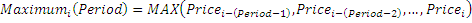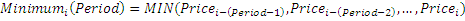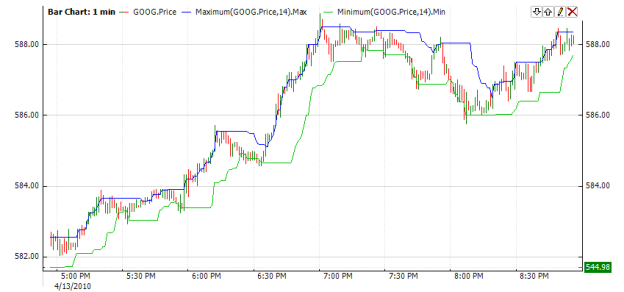﻿Maximum Minimum

# Maximum Minimum

Indicator Maximum calculates the largest value of input series on certain period. Accordingly, indicator Minimum calculates the least value of input series on certain period.CalculationChart ExampleImplementation and Usage

To initialize Maximum indicator use one of the following constructors:

Maximum – sets default Period = 14

Maximum(Int32) – sets period

To initialize Minimum indicator use one of the following constructors:

Minimum – sets default Period = 14

Minimum(Int32) – sets period

Use

Max - property for Maximum and

Min - property for Minimum

to get the current values of the indicators.Example
C#
``` 1// Create new instance
2max = new Maximum(20);
3max.HistoryCapacity = 2;
4
5min = new Minimum(30);
6min.HistoryCapacity = 2;
7